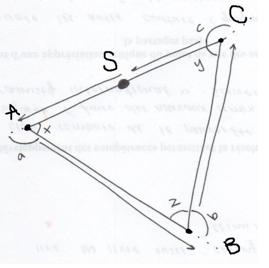#### You may also like### I'm Eight

Find a great variety of ways of asking questions which make 8.### Let's Investigate Triangles

Vincent and Tara are making triangles with the class construction set. They have a pile of strips of different lengths. How many different triangles can they make?### Noah

Noah saw 12 legs walk by into the Ark. How many creatures did he see?

# Walking Round a Triangle

##### Age 5 to 7Challenge Level

How much was turned?

Is it always the same and (the big question) - why?

There was a lot of confusion about the angles involved and most solutions that were sent in indicated $180$ degrees. This number of degrees is of course involved in what we know of triangles. However, when going around a triangle we do not turn the internal angle but $180$ minus the internal angle.

The solution sent in by a pupil from TNT school in Canada does quite well in showing this.

The triangle on which the ladybird walks has three arbitrary angles x, y, and z whose sum is $180$ degrees. The ladybird starts at S facing point A. Once it reaches A it turns an angle "a" and now faces point B. It does the same at point B and C (turning an angle "b" and face C;
turn an angle "c" and face A respectively). The sums of angles a and x, c and y, b and z are each $180$ degrees. By adding: $(a+x) + (y+c) + (z+b) =$ $540$ degrees and subtracting x,y and $z = 360$ degrees so the ladybird turns $360$ degrees.This would be the same for any triangle because this is a triangle with unspecified angles.

The other solution that tried to answer all the three questions was from Alexis who goes to Claremont Fan Court School, who goes on to say;

The Ladybird turns $360$ degrees in total. This is because in order to get back to the direction you were facing, you have to turn around $360$ degrees however many facings you go around. Ergo, this is true for any triangle, or any regular polygon for that matter.

Well done the two of you.